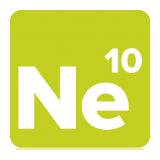Project Ne10 An open, optimized software library for the ARM architecture.
Modules
Here is a list of all modules:
[detail level 12]
 ▼Math Functions An assortment of vector/matrix math operations Vector Addition Matrix Addition Vector Subtraction (w[i] = u[i] - v) Vector Subtraction From (w[i] = v - u[i]) Matrix Subtraction Vector Multiplication Vector Multiply-Accumulate Matrix Multiplication Matrix-Vector Multiplication Vector Division Vector Assignment Vector Modulus (Length) Vector Normalization Vector Element-wise Absolute Value Vector Dot Product Vector Cross Product Matrix Determinant Calculation Matrix Inversion Matrix Transposition Matrix Identity Assignment ▼Signal Processing Functions An assortment of signal processing operations, including FFT/IFFT, FIR, and IIR operations Complex-to-Complex FFT (Floating & Fixed Point) Real-to-Complex FFT (Floating & Fixed Point) Finite Impulse Response (FIR) Filters Finite Impulse Response (FIR) Decimation Finite Impulse Response (FIR) Interpolation Finite Impulse Response (FIR) Lattice Filters Finite Impulse Response (FIR) Sparse Filters Infinite Impulse Response (IIR) Lattice Filters ▼Image Processing Functions An assortment of image processing operations, including image resize and rotation operations Image Box Filter (Blur) Image Resize Image Rotation ▼Physics Functions An assortment of collision detection operations, including AABB, relative velocity, and contact impulse calculations Collision Detection